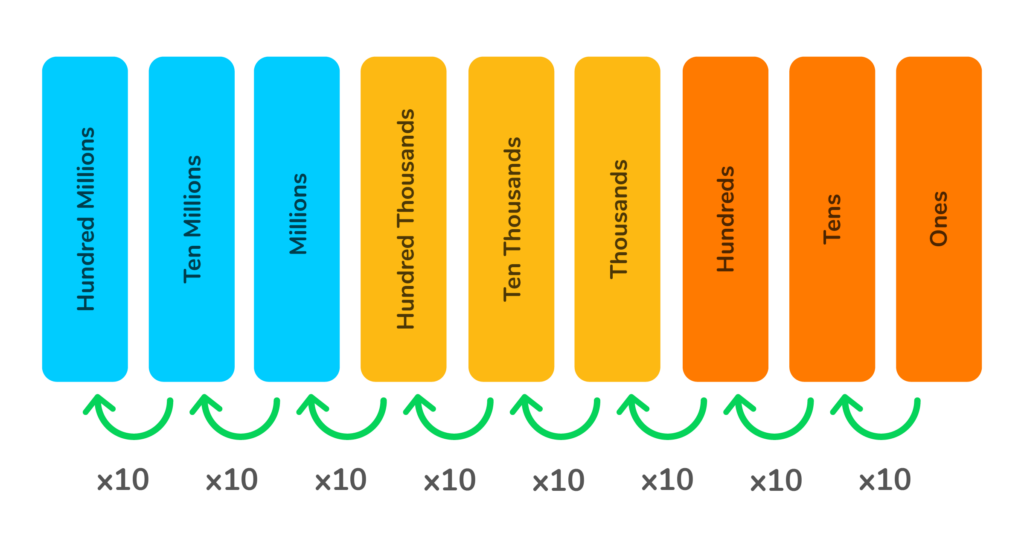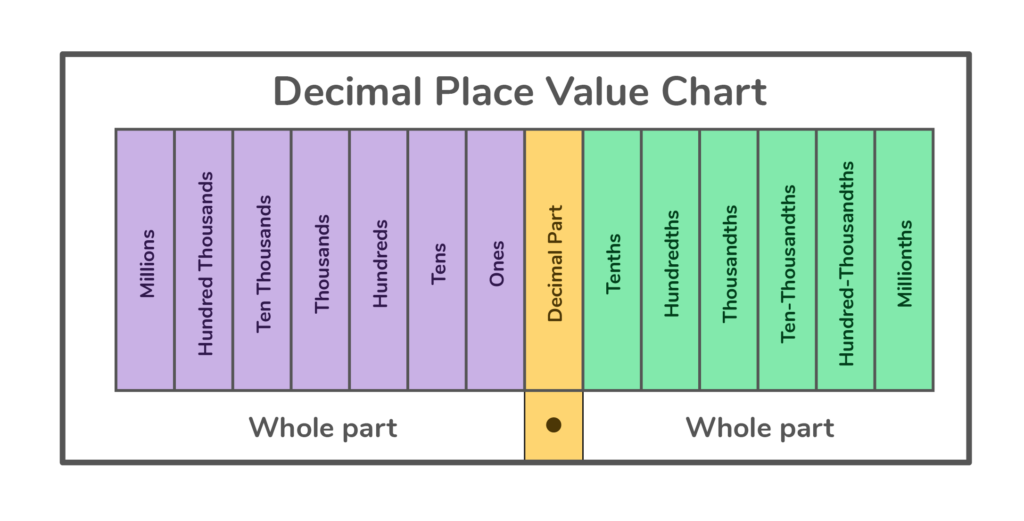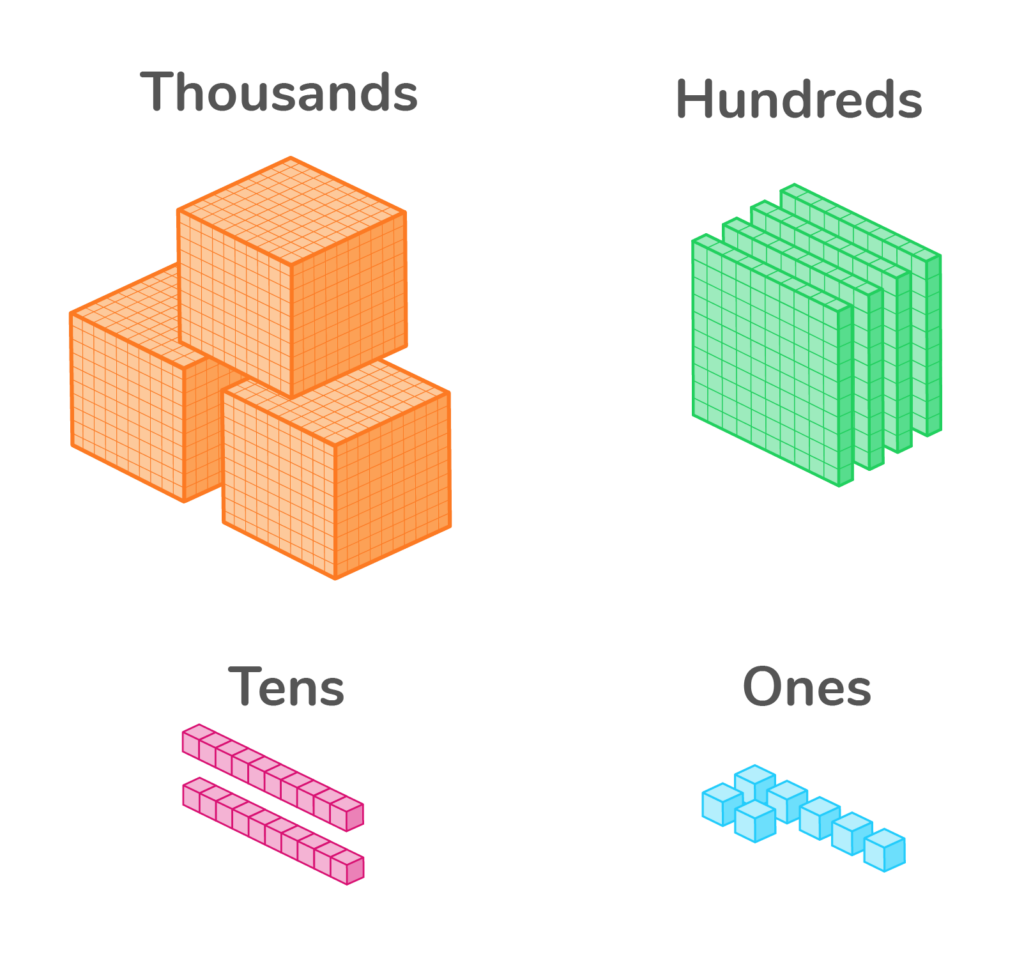# What is Place Value?

Learn what place value is and have a go at some questions!Author
Katie WickliffPublished
October 25, 2023# What is Place Value?

Learn what place value is and have a go at some questions!Author
Katie WickliffPublished
Oct 25, 2023# What is Place Value?

Learn what place value is and have a go at some questions!Author
Katie WickliffPublished
Oct 25, 2023

Key takeaways

• Place value is based on a pattern of tens.
• Every digit in a number has a place value.
• Place value charts and base ten blocks help students visualize place value.

In math, we rely on patterns to help us make sense of our numerical world. In fact, the foundation of our entire number system is place value, which is based on a pattern of tens. Learning the place value of numbers will help your student become confident at reading numbers.

## What is Place Value?Every digit in a number is assigned a place value. Basically, place values determine the worth of digits in a particular number.  Since our single digit numbers are only 1-9, we need place value to show numbers 10 and higher. Each place represents ten times the value of the position to the right.

To better understand place value, let’s look at examples with two numbers: 356 and 5,630.

The place value of 3 in the number 356 is 300. However, the place value of 3 in the number 5,630 is 30. In both numbers, the digit 3 is the same–but its value changes because of its position (or place) in the number.

Place value is also called a base ten system or a decimal system.

## Place Value ChartStudents learn place value much easier when they use a place value chart. This helpful table helps find place values for each digit in a number. As students practice identifying place value using a place value chart, they’ll develop stronger number sense. Students can practice using a chart like the one below or head to our engaging math app for more place value practice.

Eventually, students will be able to look at a number and quickly determine the place value of each digit.As this place value chart shows, the value of a digit decreases as we move right to left, and the value of a digit increases as we move left to right.

Let’s take the number 425,123 and use the chart to determine the place value for each digit.

4 is in the hundred thousands place and has a place value of 400,000.

2 is in the ten thousands place and has a place value of 20,000.

5 is in the thousands place and has a place value of 5,000.

1 is in the hundreds place and has a place value of 100.

2 is in the tens place and has a place value of 20.

3 in the ones places and has a place value of 3.

## Decimal Place Value ChartA decimal place value chart helps students find place values for digits in decimal numbers. A decimal contains both a whole number and a fractional part. They are separated by a dot called a decimal point.

In a decimal place value chart, the digits to the left of the decimal point represent the whole number part of the decimal number. The digits to the right of the decimal point represent the fractional part of the number. Each position after the decimal point becomes 10 times smaller.Let’s use this place value chart with decimals to find the values of the digits in the decimal number 64.2653.

First, let’s look at the digits to the left of the decimal point, 64.

6 is in the tens place and has a place value of 60.

4 is in the ones place and has a place value of 4.

Now, let’s look at the digits to the right of the decimal point, 2653.

2 is in the tenths place and has a place value of 0.2.

6 is in the hundredths place and has a place value of 0.06.

5 is in the thousandths place and has a place value of 0.005.

3 is in the ten-thousandths place and has a place value of 0.0003.

## Place Value Definition vs. Face Value DefinitionThe face value of a digit is how much the digit is actually worth. In contrast, we’ve learned that the place value of a digit is based on its position within the number.

For example, the face value of the digit 2 in the number 524 is 2.

The place value of the digit 2 in the number 524 is 20 (since the 2 is in the tens place).

## Place Value and Base Ten BlocksBase ten blocks, also called place value blocks, are another useful tool to show place value and help students write numbers in expanded forms. By using base ten blocks, students can easily visualize a number’s size.

Base ten blocks come in four types: unit blocks represent ones, rods represent tens, flats show hundreds, and cubes show thousands.## Practice problems

Click on each box for the answer!

5 is in the thousands place.

7 is in the tenths place and has a value of 0.7

5

3,496The place value of a number is the value of the number in a particular position.

An example of place value is the number 7 in 783 representing 7 tens, or 70.

Place value is important because it forms the basis of our whole number system. Since our single digit numbers only go up to 9, we need place value to show larger numbers.

Place value increases as it moves to the left.Lesson credits

Katie Wickliff

Katie holds a master’s degree in Education from the University of Colorado and a bachelor’s degree in both Journalism and English from The University of Iowa. She has over 15 years of education experience as a K-12 classroom teacher and Orton-Gillingham certified tutor. Most importantly, Katie is the mother of two elementary students, ages 8 and 11. She is passionate about math education and firmly believes that the right tools and support will help every student reach their full potential.

Katie Wickliff

Katie holds a master’s degree in Education from the University of Colorado and a bachelor’s degree in both Journalism and English from The University of Iowa. She has over 15 years of education experience as a K-12 classroom teacher and Orton-Gillingham certified tutor. Most importantly, Katie is the mother of two elementary students, ages 8 and 11. She is passionate about math education and firmly believes that the right tools and support will help every student reach their full potential.# Are you a parent, teacher or student?

Are you a parent or teacher?

## Hi there!

Book a chat with our team